# Olympiad Test: Exponents And Powers - 2

## 10 Questions MCQ Test Mathematical Olympiad Class 8 | Olympiad Test: Exponents And Powers - 2

Description
Attempt Olympiad Test: Exponents And Powers - 2 | 10 questions in 30 minutes | Mock test for Class 8 preparation | Free important questions MCQ to study Mathematical Olympiad Class 8 for Class 8 Exam | Download free PDF with solutions
QUESTION: 1

###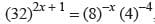the value of x will be:

Solution:

(2)5 = 32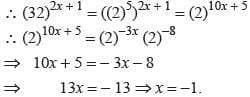QUESTION: 2

### By what number (23)-3 should be multiplied so that the product becomes (69)-1?

Solution: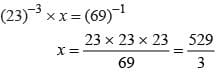QUESTION: 3

###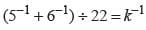then k will be equal to:

Solution: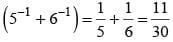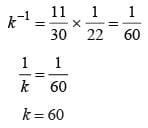QUESTION: 4

The distance between earth and the sun is 15 ×107 km. Express this distance in metres.

Solution:

Distance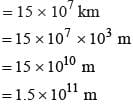QUESTION: 5

Simplify :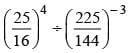Solution: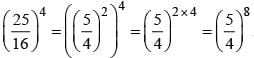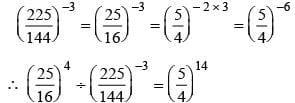QUESTION: 6

(6 + 3) × 9-1 ÷ 3-1 + 2-2 =

Solution:

Here (6 + 3) × 9-1 ÷ 3-1 + 2-2
= 9 × 9-1 ÷ 3-1 + 2-2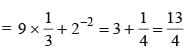QUESTION: 7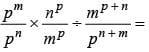m ≠ 0, p ≠ 0 and m = n = p

Solution:

We have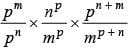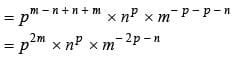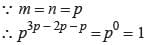QUESTION: 8

The size of a plant cell is 0.00001275 m. Express this distance in standard form.

Solution:

Size of plant cell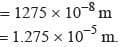QUESTION: 9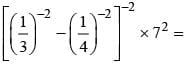Solution: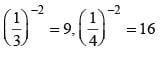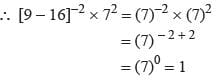QUESTION: 10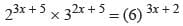then x =

Solution:

Here 23x + 5 × (3)2x + 5
= (2)3x + 2 × (3) 3x + 2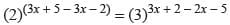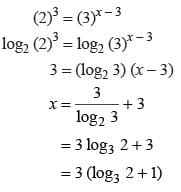Use Code STAYHOME200 and get INR 200 additional OFF Use Coupon Code